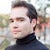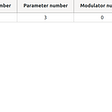# CoDEVIANT #18 (10/5/20) — Sprinkles on Top!

`function moveElementToEnd(array, toMove) { let arr = [] arr = array.filter(el => {  if(el !== toMove) {  return el  } })let counter = array.length - arr.lengthfor(let i = 0; i < counter; i++) {  arr.push(toMove)} return arr}`
`let arr = []`
`let arr = []arr = array.filter(el => {  if(el !== toMove) {     return el  }})`
`let counter = array.length - arr.length`
`for(let i = 0; i < counter; i++) {  arr.push(toMove)}return arr`
`function moveElementToEnd(array, toMove) { let soughtValues = [] let otherValues = [] array.forEach(el => {  if(el === toMove) {   soughtValues.push(el)  } else {   otherValues.push(el)  } }) return otherValues.concat(soughtValues)}`
`let soughtValues = []let otherValues = []`
`array.forEach(el => {  if(el === toMove) {   soughtValues.push(el)  } else {   otherValues.push(el)  } })`
`return otherValues.concat(soughtValues)`
`function moveElementToEnd(array, toMove) { let a = 0 let z = array.length - 1  while(a < z) {  while(a < z && array[z] === toMove) {   z --  }    if(array[a] === toMove) {   array[a] = array[z]   array[z] = toMove  }    a++ } return array}`
`let a = 0let z = array.length - 1`
`while(a < z) {   while(a < z && array[z] === toMove) {      z--   }   ...}`
`while(a < z) {   while(a < z && array[z] === toMove) {      z--   }   if(array[a] === toMove) {      array[a] = array[z]      array[z] = toMove   }}`
`while(a < z) {   while(a < z && array[z] === toMove) {      z--   }   if(array[a] === toMove) {      array[a] = array[z]      array[z] = toMove   }   a++}`
`function moveElementToEnd(array, toMove) { let a = 0 let z = array.length - 1  while(a < z) {  while(a < z && array[z] === toMove) {   z --  }    if(array[a] === toMove) {   array[a] = array[z]   array[z] = toMove  }    a++ } return array}`

--

--

--

is a web developer, opera singer, actor, and lover of cats. (adrian-rosales.tech)

Love podcasts or audiobooks? Learn on the go with our new app.

## Use Cypress to test AWS Amplify Apps with Authentication## 【鬼弒訊】▷線上看小鴨完整版~2020《 Host》~看电影完整版 — 1080P## React: Building an accessible FAQ componentis a web developer, opera singer, actor, and lover of cats. (adrian-rosales.tech)

## How To Install HomeBridge on Portainer## Play Soundfonts with Timidity in Ubuntu## Animal Part II: Plastic Forms